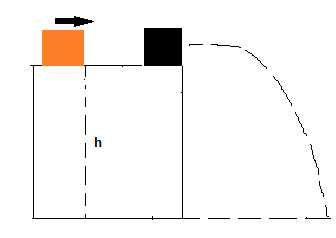# In the figure, a 3.7 kg box of running shoes slides on a horizontal frictionless table and...

## Question:

In the figure, a 3.7 kg box of running shoes slides on a horizontal frictionless table and collides with a 1.9 kg box of ballet slippers initially at rest on the edge of the table, at height h = 0.68 m. The speed of the 3.7 kg box is 3.5 m/s just before the collision. If the two boxes stick together because of packing tape on their sides, what is their kinetic energy just before they strike the floor?## Energy conservation Principle:

The energy conservation principle consists of a law that states that if no external forces act on the system, the total energy of the system should conserve. Energy is not created nor destroyed, it only transforms. This means that a system may present energy transformations, but the overall total energy of the system will remain the same. The total energy of a system can be determined by the sum of the total kinetic and potential energy of the system.

$$E_t=K_e+U_e\\ E_t=0.5mv^2+mgh$$

As the first step, we need to determine the velocity the two boxes will attain after colliding. We can use momentum conservation to determine this new velocity before they leave the table.

momentum conservation principle:

{eq}p_i=p_f\\ (m_1v_1)=(m_1+m_2)v_2\\ v_2=\dfrac{m_1v_1}{m_1+m_2}\\ v_2=\dfrac{3.7\times3.5}{3.7+1.9}\\ v_2=2.31\ m/s {/eq}

The total kinetic energy that the 2-box system will possess will consist of the total kinetic energy and potential energy at the top of the table. The total kinetic energy will be equal to the sum of those 2 values because as no external forces act on the system, the energy will conserve.

{eq}E_i=E_f\\ K_{ei}+U_{ei}=K_{ef}\\ K_{ef}=\dfrac{1}{2}(m_1+m_2)v_2^2+(m_1+m_2)gh\\ K_{ef}=0.5(3.7+1.9)2.31^2+(3.7+1.9)\times9.8\times0.68\\ K_{ef}=52.26\ J {/eq}